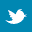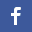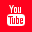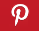Completely Solved C, C++ Programs Assignment.Quick Search Database Videos Tutorials Ebooks Forums FAQ Aboutus Household Industrial Manufacturing Service Shopping Transportation### Write a c program to check given number is perfect number or not.

 Filed Under:

 /*Write a c program to check given number is perfect number or not.*/ #include #include int main(){  int n,i=1,sum=0;  printf("\nEnter a number:-");  scanf("%d",&n);  while(i

 Definition of perfect number: Perfect number is a positive number which sum of all positive divisors excluding that number is equal to that number. For example 6 is perfect number since divisor of 6 are 1, 2 and 3.  Sum of its divisor is1 + 2+ 3 =6 Note: 6 is the smallest perfect number. Next perfect number is 28 since 1+ 2 + 4 + 7 + 14 = 28Some more perfect numbers: 496, 8128